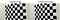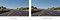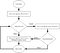# UDACITY SDCE Nanodegree: Term 1- Project 4: Advanced Lane Finding!

Through this project an algorithmic pipeline was developed capable of tracking the road lane-lines and localizing the position of the vehicle with respect to them

The goals / steps of this project were the following:

• Compute the camera calibration matrix and distortion coefficients given a set of chessboard images.
• Apply a distortion correction to raw images.
• Use color transforms, gradients, etc., to create a thresholded binary image.
• Apply a perspective transform to rectify binary image (“birds-eye view”).
• Detect lane pixels and fit to find the lane boundary.
• Determine the curvature of the lane and vehicle position with respect to center.
• Warp the detected lane boundaries back onto the original image.
• Output visual display of the lane boundaries and numerical estimation of lane curvature and vehicle position.

# Camera Calibration

The first step of this pipeline was to remove any radial/barrel distortion in the image due to deviation from rectilinear projection ( a projection in which straight lines in a scene remain straight in an image). The openCV implementation of this step starts by preparing “object points”, which will be the (x, y, z) coordinates of the chessboard corners in the world. It is assumed that the chessboard is fixed on the (x, y) plane at z=0, such that the object points are the same for each calibration image. Thus, objp is just a replicated array of coordinates, and objpoints will be appended with a copy of it every time all chessboard corners are successfully detected in the image; imgpoints will be appended with the (x, y) pixel position of each of the corners in the image plane with each successful chessboard detection. In the experiments corners were detected in 17 out of the 20 provided chessboard images.Calibration Result on Chessboard ImagesCalibration Result on Road Images

The objpoints and imgpoints arrays are used in the cv2. calibrateCamera function to extract the camera matrix (mtx) and distortion coefficients (dist). Calibration camera parameter results are saved in apickle file and are loaded when the lane tracking pipeline python file is executed. Distortion correction to test images using the cv2.undistort() function provides these results.

# Detection Pipeline

The line detection algorithms uses a combination of color and gradient thresholds to generate a binary image. Specifically, 5 different thresholding functions are used, 4 related to processing of the gradient and one for processing the saturation channel in the HLS color space. Output of each step is shown in the image below. Perspective transformation is used to obtain a birds-eye view of the line regions in order to detect them and isolate them for further processing. The transform prespective function takes as inputs an image (image), as well as the source (src) , and a percentage (perc) of the image height that controls where the source points are mapped in the output image dimensions, and hence, the final values of the destination (dst) points. The source points where selected manually by inspecting the position of the lines in the images. The perspective transform was verified by drawing the src and dst points onto a test image and its warped counterpart to verify that the lines appear parallel in the warped image.Algorithm for lane histogram detection

The process of identifying the lane-line pixels is implemented through functions lane_histogram_detection(). The former employees the sliding window approach that exhaustively searchers for line pixels, while the latter uses a faster approach that assumes the lines are located in a similar position and skips the sliding window. The sliding window process runs every 30 frames or in the event that one of the two lines is not detected in the current frame by the faster approach. After detecting the lines the polynomials fitted using the pixels corresponding to the left and right lines. Overall the pipeline stages are visualized below.

# Radius of Curvature and Vehicle Position

The radius of curvature is computed in function corrected_curvature() for both lane lines using the equation: R_curve = (1+(2Ay+B)²)^(3/2)/|2A|

The radius of curvature is computed for both lines and the average curvature is reported. The radius values are corrected by multiplying the polynomial coefficients with the term ym_per_pix which was chosen to be 30/image_height (corresponding to 30 meters per 720 pixels).

The position of the vehicle with respect to the center is computed in function find_distance_from_center() which receives as input the two line objects and image dimensions. First, the midpoint between the two lines is found, which is the average position of the bottom pixels of each line, and subtract from that the image midpoint. This is multiplied with the term ym_per_pix which was chosen to be 3.7/px_distance_between_lines (corresponding to 3.7 meters per 560 pixels). If the resulting value is negative then the vehicle is located to the right of the lane, while if it is left it is located to the left.

# Discussion

Some major issues that I faced in the pipeline implementation were related to the lines not being properly detected, especially where there where the brightness of the road increased and blended with the yellow line. I realized that I needed something more than edge processing. To address these issue, I first also transformed the image into HLS color space and isolated the saturation component, and threshold it to have a better representation of the yellow line. This allowed for detecting the line even in difficult situations. Another problem that I face was that the polynomial did not fit correctly to the line either because no lane was detected or when only a few pixels where detected resulting in a bad fit. To solve this, I average the detection over time. I implement this in the Line() class and in function update_line_status(). I also consider the measurements of the previous 10 line fits for each line and so any bad fits in the current frame do not have significant impact on the line detection and hence I achieve a smoother lane tracking.

Overall, the pipeline seems to work well on the project video and test images. However, the edge detection process used for the lane line detection can be easily confused differences in the color of the road and markings (as in the challenge video). This is something that requires more consideration. Perhaps using brightness normalization techniques and a mask that also discards the middle part of the road can help towards this direction.

PhD in Computer Engineering, Self-Driving Car Engineering Nanodegree, Computer Vision, Visual Perception and Computing

## More from Christos Kyrkou

PhD in Computer Engineering, Self-Driving Car Engineering Nanodegree, Computer Vision, Visual Perception and Computing